# Mathematics - Mathematical Analysis Double and Multiple IntegralsSource

## Introduction

Hello it's a me drifter1!

Today we continue our talk with Mathematical Analysis by getting into Double and Multiple Integrals and where they are useful!

I suggest you read my post about Integrals and maybe even the Integration Methods (that you can find in my recap), so that you understand everything better!

So, without further do, let's get straight into it!

## Recap

So, let's start simple by defining what an Integral is.

In Differential Calculus we defined derivatives/differentials. The first derivative f'(x) of a function f(x) specifies the slope/angle of the function f(x) with the axis x'x. The second derivative f''(x) then defines the "slope" of the first derivative of f(x) and so the curvature of f(x).

But, what if we have some derivative of a function and want to know the function f(x) from which we got this derivative?

That's exactly when Integrals come into the game.

The (first) integral of a function f(x) gives us a function F(x) so that F'(x) = f(x).

This can also be noted like that: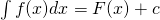quicklatex

There are a lot of properties and many techniques that you can use to find the integral of a function that I will leave out for this post, cause you can find it in my previous posts.

One more important thing is the so called Definite Integral.

A function's integral in a specific range Δx = x2 - x1.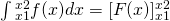quicklatex

The definite integral gives out a real value and not a function...

There are also integrals that go to infinity and so on, but I think that that's enough for understanding what I will say today! :)

## Double Integral as Plane Projection

Suppose we have a plane/surface S: z = f(x, y).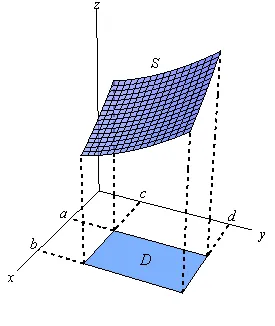Source

The projection of S into the plane xOy which is an rectangle and in an closed/ area defines a Volume: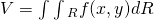quicklatex

You can understand that better if you thing of small Δx*Δy = dR areas so that: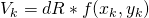Which means that the Volume of this solid object is:

V = ΣVk = Σ f(x, y)*dR

By getting the limits Δx -> 0 and Δy -> 0 this transforms to:

V = lim Σ f(x, y)*Δx*Δy =## Fubini Theorem and Properties

You might remember from Partial Derivatives that we can differentiate with x and y in any order we like. This means that we can differentiate with x and then y OR with y and then x.

So,  ∂^2f /∂y∂x = ∂^2f/∂x∂y

This same principle can also be applied in Double Integrals.

The Fubini Theorem says that:
quicklatex

And so we can (partially) integrate with any order we like, but we have to keep the same ranges for the corresponding variables x or y.

So, sometimes we might start the integration with one instead of the other variable, cause the calculations might be easier!

For example:quicklatex

Solve the "opposite" order integral!

You can see that I also added parenthesis so that it is easier to distinguish!

To get the other order we have to plot the function e^x: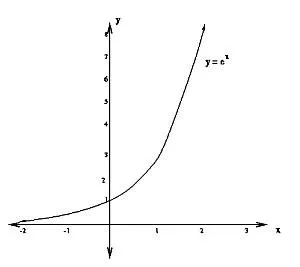Source

We are talking about the area between:

• (0, 1) in the x-axis and
• (1, e) in the y-axis, cause e^1 = e.

This is the folowing marked area: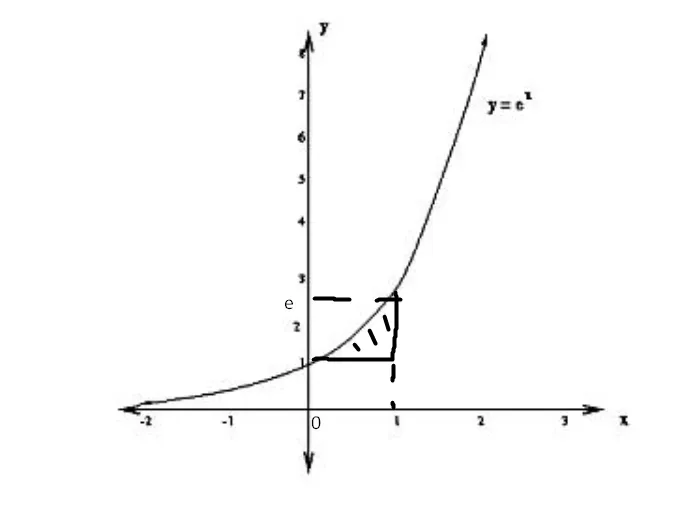e^x = y => x = lny

So, the new ranges are:

• (1, e) in the y-axis and
• (lny, 1) in the x-axis, cause x = lny

And so we can rewrite the integral like that: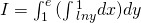quicklatex

This is the "opposite" integral. Let's now solve it!quicklatex

For lnx you actually use integration by parts, but I left it out for simplicity!

You could see that I used a lot of properties like:

• Multiplication with an constant

These are very useful and are the same as with "normal" integrals...

## Volume and Area using Double Integrals

You can already see that we use a Double Integral inside of the "area" R to calculate the volume of an function f(x, y).

But, what if f(x, y) is 1? (like in our example)

Well when setting f(x, y) = 1 we actually calculatethe Area of R.

So, the two main "equations" that you should keep in mind are: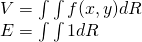quicklatex

which calculate the Volume in between of f(x, y) and the area R AND the actual area R.

## Multiple Integrals

So, to sum up now:

• A single integral is used to find "derivated" function F(x) or the Area by using the definite integrals.
• A double integral is used for finding the two-times derivated function F(x) or the Volume of f(x, y) or the area R of the range of double-integration.

The N-integral will of course find the function F(x) that was derivated N-times.

We of course also define multiple integrals for more variables, but they are mostly used in Physics problems of mass and don't have a lot more applications...and mostly used in spherical/polar coordinates and not in the Cartesian Space.

Thinking about the Double integral again we saw that by setting f(x, y) as 1 we got the Area.

By doing the same with A Triple Integral we get the Volume...

But, what does the Triple Integral of f(x, y, z) represent?

Well, here we go again...

There are more dimensions than the 3 that we can perceive, and so the Triple-Integral might represent something higher then the Volume!

We define points, lines, areas, volumes, but is there more?

I will end this here, so that we don't get more mind-f*cked today xD

I guess you have a lot of stuff to search for now :P

And this is actually it for today and I hope that you enjoyed it!

Next time we will get into Surface and Contour Integrals...

Bye!

H2
H3
H4
3 columns
2 columns
1 column
Join the conversation now